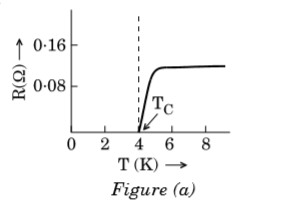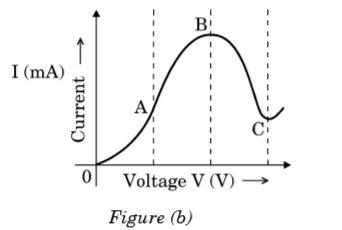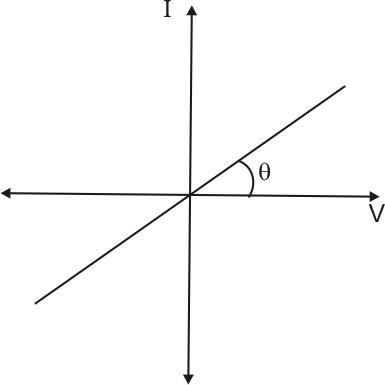# (a) Draw a graph showing the variation of current versus voltage in an electrolyte when an external resistance is also connected. (b) (i) The graph between resistance (R) and temperature (T) for Hg is shown in the figure (a). Explain the behaviour of Hg near 4 K.(ii) In which region of the graph shown in the figure (b) is resistance negative and why ?a) When an external resistance is connected to an electrolyte then there is a voltage drop in the resistance so the voltage across will reduce. When we plot a graph showing the variation of current versus voltage it is a straight line with a lesser slope. It is due to the presence of external resistance which implies for flowing the same current in the circuit with external resistance require more voltage than the circuit without resistance.b)  i)Here at 4 K temperature the resistance of Hg becomes zero which is the characteristic shown by superconductors. So we can say that Hg behaves as superconductors at the temperature of 4K

ii)From the graph, it shows that from B to C resistance is negative. Here when voltage increases current is decreased which means resistance is negative.

## Related Chapters

### Preparation Products

##### Knockout NEET July 2020

An exhaustive E-learning program for the complete preparation of NEET..

₹ 15999/- ₹ 6999/-
##### Rank Booster NEET 2020

This course will help student to be better prepared and study in the right direction for NEET..

₹ 9999/- ₹ 4999/-
##### Knockout JEE Main July 2020

An exhaustive E-learning program for the complete preparation of JEE Main..

₹ 12999/- ₹ 6999/-
##### Test Series NEET July 2020

Take chapter-wise, subject-wise and Complete syllabus mock tests and get in depth analysis of your test..

₹ 4999/- ₹ 2999/-